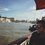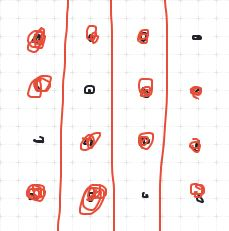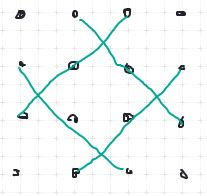# Right Angled Triangle

Hello,

I have come across a problem that I don't have an elegant solution for...

The question is: Given a square 4 x 4 grid of dots, how many right angled triangles can you create by selecting three dots?

You could of course use a brute force method. I have considered permutations / combinations, but ran into some difficulties of eliminating solutions. I've considered approaching it through a symmetrical approach as well.

Any help on the problem will be very helpful!Note by Jae Joon Chang
7 months, 4 weeks ago

This discussion board is a place to discuss our Daily Challenges and the math and science related to those challenges. Explanations are more than just a solution — they should explain the steps and thinking strategies that you used to obtain the solution. Comments should further the discussion of math and science.

When posting on Brilliant:

• Use the emojis to react to an explanation, whether you're congratulating a job well done , or just really confused .
• Ask specific questions about the challenge or the steps in somebody's explanation. Well-posed questions can add a lot to the discussion, but posting "I don't understand!" doesn't help anyone.
• Try to contribute something new to the discussion, whether it is an extension, generalization or other idea related to the challenge.
• Stay on topic — we're all here to learn more about math and science, not to hear about your favorite get-rich-quick scheme or current world events.

MarkdownAppears as
*italics* or _italics_ italics
**bold** or __bold__ bold
- bulleted- list
• bulleted
• list
1. numbered2. list
1. numbered
2. list
Note: you must add a full line of space before and after lists for them to show up correctly
paragraph 1paragraph 2

paragraph 1

paragraph 2

[example link](https://brilliant.org)example link
> This is a quote
This is a quote
    # I indented these lines
# 4 spaces, and now they show
# up as a code block.

print "hello world"
# I indented these lines
# 4 spaces, and now they show
# up as a code block.

print "hello world"
MathAppears as
Remember to wrap math in $$ ... $$ or $ ... $ to ensure proper formatting.
2 \times 3 $2 \times 3$
2^{34} $2^{34}$
a_{i-1} $a_{i-1}$
\frac{2}{3} $\frac{2}{3}$
\sqrt{2} $\sqrt{2}$
\sum_{i=1}^3 $\sum_{i=1}^3$
\sin \theta $\sin \theta$
\boxed{123} $\boxed{123}$

## Comments

Sort by:

Top Newest

@Jae Joon Chang - Here is the solution-

Basically what we are looking for is the number of sets of 3 points within 16 points minus the sets of 3 points that lie on the same line, or in other words are collinear.

The number of sets of 3 points within the 4x4 grid is $C^{16}_{3} = 560$

Now, on each vertical and horizontal line, there are 4 ways that you can choose 3 collinear points (Proof given at the end)

There are 4 vertical lines, and 4 horizontal lines, so the number of sets here is $4 \times 4 \times 2 = 32$

The grid has two main diagonals, and four minor diagonals (Shown in an image at the end)

Again, there are 4 ways that 3 collinear points can be chosen on the main diagonals, and 1 way on each of the minor diagonal lines.

This means, that the number of sets on the diagonals is $2 \times 4 + 4 = 12$

Thus, the answer is $560-32-12 = \boxed{516 \text{ triangles}}$

Images -These are the 4 ways you can choose a set of 3 collinear points on a lineThe green lines are the minor diagonals

Sorry for bad artistic skills :)

- 7 months, 3 weeks ago

Log in to reply

@Percy Jackson - thanks for your solution. I think the 516 triangles are simply triangles, whereas the question wanted to find the number of potential right angled triangles you can get.

A friend of mine ran a C++ programme finding three points that satisfies Pythagoras' Theorem and it returned with 200 right angled triangle.

I like the idea of eliminating collinear points, but I'm not too sure on how you could look to eliminate points that create a scalene triangle.

- 7 months, 3 weeks ago

Log in to reply

Oh! Right angled triangles! I missed that part. Yes, then it would be difficult to eliminate all other triangles which are not right angled...Can you share the C++ code with me if its possible?

- 7 months, 3 weeks ago

Log in to reply

apologies for the formatting, but this is what my friend had put in.

IN: import itertools

IN: def is_right(a,b,c): ab2 = (a-b)(a-b) + (a-b)(a-b) ac2 = (a-c)(a-c) + (a-c)(a-c) bc2 = (b-c)(b-c) + (b-c)(b-c) s = sorted((ab2, ac2, bc2)) return (s + s == s and a != b and a != c and b != c)

IN: def righttriangles (x, y): return {frozenset((a, b, c)) for (a, b, c) in itertools.product( itertools.product(range(x), range(y)), itertools.product(range(x), range(y)), itertools.product(range(x), range(y)) ) if isright(a, b, c)}

IN: len(right_triangles(4, 4))

- 7 months, 3 weeks ago

Log in to reply

ok, thanks!

- 7 months, 3 weeks ago

Log in to reply

I ran a simple python program and I got only 32 lol

- 6 months, 2 weeks ago

Log in to reply

  1 2 3 4 5 6 7 8 9 10 11 12 13 14 15 16 17 18 19 20 def dist(x1,y1,x2,y2): return ((x1-x2)**2+(y1-y2)**2)**0.5 count=0 for x1 in range(4): for y1 in range(4): for x2 in range(4): for y2 in range(4): for x3 in range(4): for y3 in range(4): if dist(x1,y1,x2,y2)!=0 and dist(x1,y1,x3,y3)!=0 and dist(x2,y2,x3,y3)!=0: if dist(x1,y1,x2,y2)**2+dist(x1,y1,x3,y3)**2==dist(x2,y2,x3,y3)**2: count+=1 elif dist(x1,y1,x2,y2)**2+dist(x2,y2,x3,y3)**2==dist(x1,y1,x3,y3)**2: count+=1 elif dist(x3,y3,x2,y2)**2+dist(x1,y1,x3,y3)**2==dist(x2,y2,x1,y1)**2: count+=1 print(count) 

- 6 months, 2 weeks ago

Log in to reply

It outputted 192 but since every triangle was counted six times, the answer according to me should be surprisingly just 32, please tell me if the program is wrong ( pretty sure it is given the number solutions your friend predicted )

- 6 months, 2 weeks ago

Log in to reply

I think you've got precision problems with floats. Rerun it with d2 instead of dist, where you don't take square roots (and drop the squares from the if statements in the loop). I did that myself, and I got 200.

The answer has to be at least 144; pick a point to be the right-angle point, and then pick a point on the vertical line and a point on the horizontal line from that point. There are $16 \cdot 3 \cdot 3$ choices. And that ignores triangles like $(0,0),(1,1),(2,0).$

- 6 months, 1 week ago

Log in to reply

Oh Thanks lol that was silly, I still got a problem though, I tried your method and got 1200 as expected but if I went with four-five digits of accuracy (with the square root) I get 1080 ( goes to 200 and 180 ) and python does have an accuracy to over ten digits, if anything I should be getting extraneous answers, how is it coming less?

- 6 months, 1 week ago

Log in to reply

Your "if" conditions at the end of the program are harder to satisfy--maybe your floats are not quite equal but are very close to equal.

- 6 months, 1 week ago

Log in to reply

I multiplied the floats by 1000,10000 and took the integral part, that’s what I meant by four-five digits accuracy, the same answer came even when I went to 14 digits of accuracy and over 14 digits reduced the number of triangles, so from 3 - 14 digits of accuracy it kept giving me 1080 never 1200, if the problem was with floats why isn’t this working. Why are those 20 triangles elusive to this program?

- 6 months ago

Log in to reply

Never mind found the reason, (0,0),(0,2),(3,0) was one of the triangles missed, the sides squared went to 13 but the hypotenuse squared went to 12.99999999999999, I should have rounded up

- 6 months ago

Log in to reply

×

Problem Loading...

Note Loading...

Set Loading...